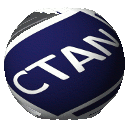# Directory `graphics/metapost/contrib/macros/matlab`

```matlab.mp ------- Metapost data plotting in matlab style

Yang Yang yangyang@juggler.ucsd.edu

March 3 2005

Matlab has powerful data plotting ability, but one annoying problem
prevents it from being perfect: when you increase the linewidth, the
dotted line size stays the same, so the plotted line no longer looks
like a dotted line.

another problem is matlab bug: it oftern cuts away some curves and
captions.

plus, matlab is expensive. on the other hand, the free gnuplot also
doesn't produce very satisfactory visual effects.

I got accustomed to matlab's easy interface. so I want to integerate
the powerful graphic function of Metapost and the easy interface of
Matlab. so I wrote this script matlab.mp. this is based on graph.mp, I
just collected the data into 2-D arrays, and remembered the line
styles, so it's easy to plot out legends.

FEATURES:
all kinds of line color, width, style (dotted/dashed, etc), and
markers. you can easily expand this by modifying the code.

easy interface. very similar to matlab

full power of latex in caption/legends, by using the latexmp package.

```

## metapost-matlab – METAPOST data plotting in Matlab style

Matlab has powerful data plotting ability, but one annoying problem prevents it from being perfect: when you increase the linewidth, the dotted line size stays the same, so the plotted line no longer looks like a dotted line.

Another problem is Matlab's bugs: it often cuts away some curves and captions. And, of course, Matlab is expensive. On the other hand, the free Gnuplot doesn't produce very satisfactory visual effects either.

The author got accustomed to Matlab's easy interface, and wanted to integerate the powerful graphic functions of with the interface of Matlab. The outcome was matlab.mp. It is based on graph.mp (part of the distribution). The package collects data into 2D arrays, and remembers the line styles, so it's easy to plot out legends. The package features:

– All kinds of line colour, width, style (dotted/dashed, etc), and markers (the code may easily be expanded);
– Easy interface, very similar to Matlab;
– The full power of is available in captions and legends, by using the latexmp package.

 Package metapost-matlab Licenses The LaTeX Project Public License Maintainer Yang Yang Topics Graphics plot See also metapostlatexMP